# Integral hyperbolic cosine

(diff) ← Older revision | Latest revision (diff) | Newer revision → (diff)

The special function defined, for real, bywhere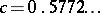is the Euler constant and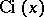is the integral cosine. The integral hyperbolic cosine can be represented by the series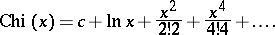Sometimes it is denoted by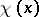.

For references, see Integral cosine.

This function, which is seldom used because of its relation with the cosine integral, is also called the hyperbolic cosine integral. It can, of course be defined (as above) for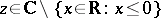.
One has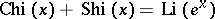, where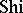is the integral hyperbolic sine and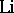is the integral logarithm.